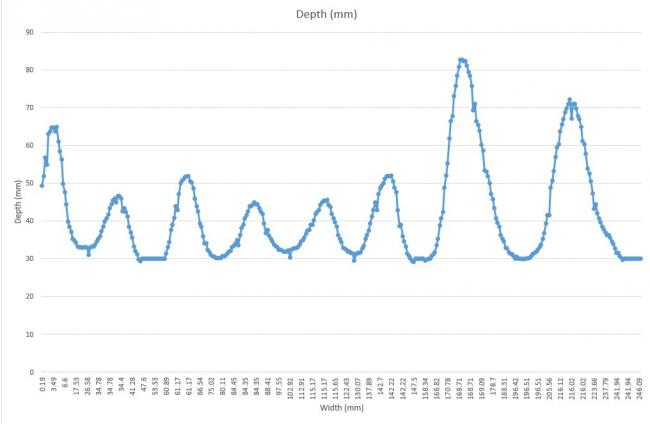#### Howdy, Stranger!

We are about to switch to a new forum software. Until then we have removed the registration on this forum.

# Create a graph (live-feed or after data collection) of sensor data from an Arduino serial port

edited November 2015 in Arduino

Hi,

I have a code which takes data from 2 sensors that is incoming through the serial port of an Arduino and outputs it into a .csv file. This data is received as a float of the format "y, x" which is then delimited/split by the use of the comma and put into an array. The "y" data is a depth in mm measurement and the "x" is a correlating distance moved in mm.

I have been trying to get the code to output either a live-feed line graph or a line graph after the file is written with data "x" on the x-axis and "y" on the y-axis.

My experience with Processing is very limited and I have tried with no luck to adapt pretty much all of the examples I could find on the various forums and tutorials. Any help that could be offered would be very gratefully received, I don't mind if the solution is live-feed or after the .csv is written just having a graph out would be great.

Below I have shown a plot I did manually in excel to show the kind of output I am trying to get as well as the code I have got already which I have annotated on to try to make understanding my process as pain-free as possible.``````import processing.serial.*;
Serial myPort; //creates a software serial port
Table dataTable; //table where values are stored
Table table;

int numReadings = 500; //number of readings from sensors until .csv file is written

String val;
String fileName;

void setup()
{
String portName = Serial.list(); //defines COM port

myPort = new Serial(this, portName, 9600); //port setup, 9600 baud rate

table = new Table();

//table.addColumn("id"); //id number for incoming data starting

//the following adds columns for time. (currently not used)

//the following adds columns for each sensor set. Change for sensors and keep in correct order.

}

void serialEvent(Serial myPort){

try {
val = myPort.readStringUntil('\n'); //The newline separator separates each Arduino loop and so collection of data.
if (val!= null) { //Verifies reading
val = trim(val); //gets rid of any whitespace or Unicode nonbreakable space
float sensorVals[] = float(split(val, ',')); //parses the packet from Arduino and places the float values into the sensorVals array.

//following not used at present
//newRow.setInt("id", table.lastRowIndex());//record an id for readings

//record time stamp
//newRow.setInt("year", year());
//newRow.setInt("month", month());
//newRow.setInt("day", day());
//newRow.setInt("hour", hour());
//newRow.setInt("minute", minute());
//newRow.setInt("second", second());

//record sensor information. Make sure same as sensor column names.
newRow.setFloat("Depth", sensorVals);
newRow.setFloat("Width", sensorVals);

//saves the table as a csv in the same folder as the sketch every numReadings.
{
fileName = str(year()) + str(month()) + str(day()) + str(table.lastRowIndex()); //filename is of the form year+month+day+readingCounter
saveTable(table, fileName + ".csv"); //saves the data as a .csv
}
}
}
catch(RuntimeException e) {//catches errors
e.printStackTrace();
}

}

void draw () {

}
``````

Sam

Tagged:

Here is an example that draws such a graph. It gets more complex if you have to scale it automatically to fit the screen, but maybe it's a starting point.

``````Table table;

void setup() {
size(1000, 500);

// create table
table = new Table();
}

void draw() {
background(255);

// iterate over table-rows
for (int i =0; i<table.getRowCount(); i++) {

TableRow row = table.getRow(i);
// draw circle
float x = row.getFloat("Width")*5;
float y = row.getFloat("Depth")*5;
ellipse(x, y, 10, 10);

// lines (connect this point with the last one)
if (i > 0) {
TableRow lastRow = table.getRow(i-1);
float lastX = lastRow.getFloat("Width")*5;
float lastY = lastRow.getFloat("Depth")*5;

line(lastX, lastY, x, y);
}
}
}

void mousePressed() {